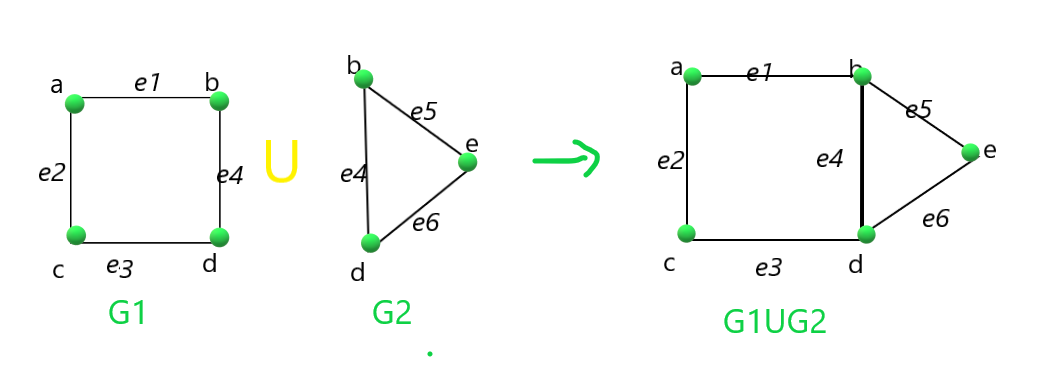# Union and Intersection of two Graphs

• Difficulty Level : Easy
• Last Updated : 21 Apr, 2021

Given two graphs G1 and G2, the task is to find the union and intersection of the two given graphs, i.e. (G1 ∪ G2) and (G1 ∩ G2).

Examples:

Input: G1 = { (“e1”, 1, 2), (“e2”, 1, 3), (“e3”, 3, 4), (“e4”, 2, 4) }, G2 =  = { (“e4”, 2, 4), (“e5”, 2, 5), (“e6”, 4, 5) }
Output:
G1 union G2 is
e1 1 2
e2 1 3
e3 3 4
e4 2 4
e5 2 5
e6 4 5

G1 intersection G2 is
e4 2 4
Explanation:
Union of the graphs G1 and G2:Intersection of the graphs G1 and G2:

Approach: Follow the steps below to solve the problem:

• Define a function, say Union(G1, G2), to find the union of the G1 and G2:
• Initialize a map, say added, that stores if an edge is already been added or not.
• Iterate over the edges of the graph G1 and push all the edges in a graph, say G, and mark all the edges visited in added.
• Now, again traverse over the edges of the graph G2 and push the edge in the G if the edge is not already been added, and then mark the edge added in the map added.
• Define a function say Intersection(G1, G2) to find the Intersection of the G1 and G2:
• Initialize a map, say added , that stores if an edge is already been added or not.
• Traverse over the edges of the graph G1 and marked all the edges visited in the map added.
• Now, again traverse over the edges of the graph G2 and push the edge in the graph G, if the edge is already been added. Then, mark the edge added in the map.
• Now, print the graphs obtained after the function call of Union(G1, G2) and Intersection(G1, G2).

Below is the implementation of the above approach:

## C++14

 `// C++ program for the above approach ` `#include ` `using` `namespace` `std; ` ` `  `// Function to find union of two graphs ` `void` `find_union( ` `    ``vector > G1, ` `    ``vector > G2) ` `{ ` `    ``// Stores an edge of the graph G1 ` `    ``map > added; ` ` `  `    ``// Stores the unioun graph G1 ` `    ``vector > G; ` ` `  `    ``// Iterate over the edges ` `    ``// of the graph G1 ` `    ``for` `(``auto` `p : G1) { ` ` `  `        ``string a = get<0>(p); ` ` `  `        ``// Get the edges ` `        ``int` `b = get<1>(p); ` `        ``int` `c = get<2>(p); ` ` `  `        ``// Insert the current ` `        ``// edges into graph G ` `        ``G.push_back( ` `            ``make_tuple(a, b, c)); ` `        ``added[a] = { b, c }; ` `    ``} ` ` `  `    ``// Iterate over the edges ` `    ``// of the graph G1 ` `    ``for` `(``auto` `p : G2) { ` ` `  `        ``string a = get<0>(p); ` `        ``int` `b = get<1>(p); ` `        ``int` `c = get<2>(p); ` ` `  `        ``pair<``int``, ``int``> x = { b, c }; ` `        ``pair<``int``, ``int``> y = { c, b }; ` ` `  `        ``// If either edge x or ` `        ``// y is already added ` `        ``if` `(added[a] == x || added[a] == y) ` `            ``continue``; ` ` `  `        ``// Otherwise ` `        ``G.push_back(make_tuple(a, b, c)); ` `    ``} ` ` `  `    ``// Print the unioun ` `    ``cout << ``"G1 union G2 is\n"``; ` ` `  `    ``for` `(``auto` `p : G) { ` ` `  `        ``string a = get<0>(p); ` `        ``int` `b = get<1>(p); ` `        ``int` `c = get<2>(p); ` `        ``cout << a << ``" "` `<< b << ``" "` `             ``<< c << endl; ` `    ``} ` `} ` ` `  `// Function to find intersection of two graphs ` `void` `find_intersection( ` `    ``vector > G1, ` `    ``vector > G2) ` `{ ` `    ``// Stores an edge ` `    ``map > added; ` ` `  `    ``// Stores the graph of intersection ` `    ``vector > G; ` ` `  `    ``// Iterate over edges of graph G1 ` `    ``for` `(``auto` `p : G1) { ` `        ``string a = get<0>(p); ` `        ``int` `b = get<1>(p); ` `        ``int` `c = get<2>(p); ` ` `  `        ``added[a] = { b, c }; ` `    ``} ` ` `  `    ``// Iterate over edges of graph G2 ` `    ``for` `(``auto` `p : G2) { ` ` `  `        ``string a = get<0>(p); ` `        ``int` `b = get<1>(p); ` `        ``int` `c = get<2>(p); ` ` `  `        ``pair<``int``, ``int``> x = { b, c }; ` `        ``pair<``int``, ``int``> y = { c, b }; ` ` `  `        ``// If either edge x or ` `        ``// y is already added ` `        ``if` `(added[a] == x || added[a] == y) ` `            ``G.push_back(make_tuple(a, b, c)); ` `    ``} ` ` `  `    ``// Print the graph G ` `    ``cout << ``"G1 intersection G2 is\n"``; ` ` `  `    ``for` `(``auto` `p : G) { ` ` `  `        ``string a = get<0>(p); ` `        ``int` `b = get<1>(p); ` `        ``int` `c = get<2>(p); ` ` `  `        ``cout << a << ``" "` `<< b ` `             ``<< ``" "` `<< c << endl; ` `    ``} ` `} ` ` `  `// Driver Code ` `int` `main() ` `{ ` `    ``vector > G1 ` `        ``= { make_tuple(``"e1"``, 1, 2), ` `            ``make_tuple(``"e2"``, 1, 3), ` `            ``make_tuple(``"e3"``, 3, 4), ` `            ``make_tuple(``"e4"``, 2, 4) }; ` ` `  `    ``vector > G2 ` `        ``= { make_tuple(``"e4"``, 2, 4), ` `            ``make_tuple(``"e5"``, 2, 5), ` `            ``make_tuple(``"e6"``, 4, 5) }; ` ` `  `    ``// Function call for finding the ` `    ``// Union of the given graph ` `    ``find_union(G1, G2); ` ` `  `    ``// Function call for finding the ` `    ``// Intersection of the given graph ` `    ``find_intersection(G1, G2); ` ` `  `    ``return` `0; ` `} `

Output:

```G1 union G2 is
e1 1 2
e2 1 3
e3 3 4
e4 2 4
e5 2 5
e6 4 5
G1 intersection G2 is
e4 2 4
```

Time Complexity: O(N * log(N))
Auxiliary Space: O(N)

My Personal Notes arrow_drop_up
Recommended Articles
Page :